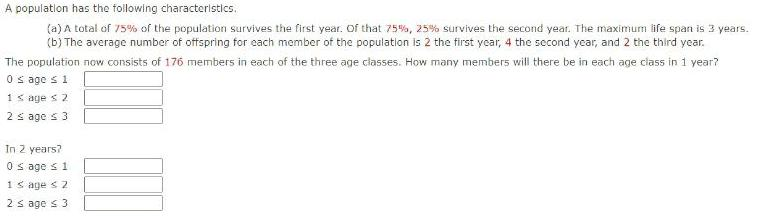Question:

# A population has the following characteristics. (a) A total

Last updated: 7/7/2022A population has the following characteristics. (a) A total of 75% of the population survives the first year. Of that 75%, 25% survives the second year. The maximum life span is 3 years. (b) The average number of offspring for each member of the population is 2 the first year, 4 the second year, and 2 the third year. The population now consists of 176 members in each of the three age classes. How many members will there be in each age class in 1 year?